## Laguerre Differential Equation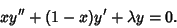(1)

The Laguerre differential equation is a special case of the more general associated Laguerre differential equation''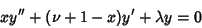(2)

with. Note that if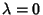, then the solution to the associated Laguerre differential equation is of the form(3)

and the solution can be found using an Integrating Factor(4)

so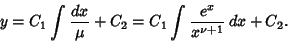(5)

The associated Laguerre differential equation has a Regular Singular Point at 0 and an Irregular Singularity at. It can be solved using a series expansion,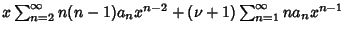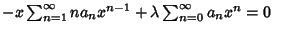(6)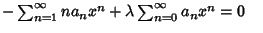(7)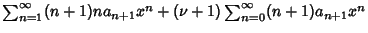(8)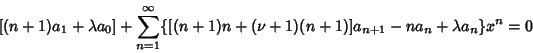(9)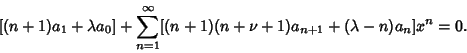(10)

This requires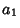(11)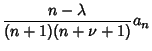(12)

for. Therefore,(13)

for, 2, ..., so(14)

Ifis a Positive Integer, then the series terminates and the solution is a Polynomial, known as an associated Laguerre Polynomial (or, if, simply a Laguerre Polynomial).